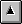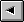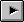## GENERAL GLOSSARYThe following section is the general word glossary for Until. All of the words are defined here.

!
```      5 trash !
```
moves 5 into the variable trash.
"
( --- 'constant-string )
"double-quote". Generate a constant string bound by a ". Character quoting using the '\' character ala C is allowed. For example:
```    " This is a string\n"
```
defines a constant string with a <newline> as its last character.
#
(ud1 --- ud2)
"sharp". Convert the next digit in ud1 to ASCII and add it to the numeric string being built. Used with <#.
#>
"sharp-greater-than". Ends a number formatting sequence. See <#.
#s
(ud --- 00)
"numbers". Convert the unsigned double number to numeric digits.
#tib
( --- 'tib )
"number-tib". This word returns the address of the Terminal Input Buffer (tib).
'
( --- 'word )
"tick". Tick looks up the next blank delimited token in the input stream in the Dictionary and leaves its address on the stack.
```       ' aword
```
leaves the word address of aword on the data stack. The operation of tick is slightly different from Forth-83 because it actually leaves the address of the word entry (WA) rather than the CFA. The effect is the same because Until's execute works that way. It is easy to go from the word address to the fields, but was not easy to go from one header field to another.
(
( --- )
"left paren". Text between the parenthesis is treated as a comment. Left parens starts a comment. A parens comment may span multiple lines.
```       ( This is a comment )
```
Use \ to comment to the end of the current line.
*
(n1 n2 --- n1*n2 )
"star". Multiply n1 times n2.
```      2 2 *
```
The result, 4, is placed on the top of the stack.
*/
(n1 n2 n3 --- quot )
"star-slash". Multiply n1 times n2, then divide by n3. (n1*n2/n3). The quotient is left on the stack.
```      3 3 2 */
```
leaves 4 on the stack.
*/mod
( n1 n2 n3 --- quot rem )
"star-slash-mod". Multiply n1 * n2, then divide by n3, leaving the quotient and remainder on the stack. (n1*n2/n3).
```      4 4 2 */mod
```
results in 8 0 on the stack. (4*4/2).
+
( n1 n2 --- n1+n2 )
"plus". Add n1 to n2. The sum is placed on the top of the stack.
```      1 2 +
```
The result, 3, is left on the top of the stack.
+!
```      10 trash +!
```
trash contains now contains 37.
+loop
( n --- )
"plus loop". The end of a do loop. The loop index is incremented by 'n' each time through the loop.
```      : xxx 10 0 do i . 2 +loop ;
```
This do loop will execute 5 times. +loop can be used only inside a word definition. See do.
,
( n --- )
"comma". Not implemented. This word compiles a 32-bit number into the dictionary.
-
( n1 n2 --- n3 )
"minus". Subtract n2 from n1 and place the result on the top of the stack. (n1-n2=n3).
```      2 1 -
```
The result, 1, is left on the stack.
-find
( 'string --- addr flag )
"minus-find". Search the dictionary for the word name in string. The return values are the address of the word and a flag. The flag values are:
``` 0=not found
1=normal
-1=immediate
```
The address is valid only when flag is non-zero. A typical usage of -find is:
```      { aword} here -find
```
The return value could be tested before further processing.
-rot
( n1 n2 n3 --- n3 n1 n2)
"minus-rot". Move the value on the top of the parameter stack to the third item.
```      1 2 3  -rot
```
becomes 3 1 2.
-trailing
"minus-trailing". Remove trailing blanks from the counted string at addr. The length, len, is adjusted accordingly. If trash contains ``This is a test ''. The stack would have 68642 17.
```      trash count -trailing
```
results in 68642 14. (Trust me, there are three trailing blanks!)
.
( n --- )
"dot". Display the number at the top of the parameter stack to the screen. This is a stack destructive operation. Note: Only base 8, 10, and 16 are supported by this operation.
."
( --- )
"dot quote". Type a string to the screen. Used in the form:
```	." This goes to the screen."
```
This word can be used only inside of a word definition. Use .( for interactive or stream messages.
.(
( --- )
"dot-parens". Type a constant string to the terminal screen. The string is terminated with a right parens. This word is used during interpretive mode only.
```      .( This is displayed.)
```
The typical usage is writing messages to the screen when compiling from a file.
.bin
( n --- )
"dot-bin". Type the 32-bit binary representation of number on the top of the stack.
.move_string
High level (addr len 'destination --- )
"dot move string". This is a variation of move_string that uses an address and length to specify the source string. destination specifies the address of the counted string that receives the source. No provision is made for overlapping strings.
.quote
( --- )
This is an internal word that should not be used. .quote is the run-time code for .".
.s
dot_s ( --- )
"dot s". Non-destructive Data Stack display to the screen. Until also displays the stack contents each time through the main loop in the outer interpreter.
.strap
High level (addr len 'destination --- )
"dot strap". end the string at addr to destination for len bytes. This word is a variation of strap.
/
( n1 n2 --- quot )
"slash". Divide n1 by n2. (n1/n2).
```      4 2 /
```
Leaves 2 on the top of the stack. There is no remainder.
```      9 2 /
```
computes to 4. Until does not deal with floored division vs. normal division. It uses the standard C division operator. And that's that!
/mod
( n1 n2 --- quotient remainder )
"slash-mod". Divide n1 by n2 leaving the quotient and remainder on the parameter stack. (n1/n2).
```      10 3 /mod
```
results in (3 1) being left on the stack.
0
( --- 0 )
"zero". Push a constant of 0 onto the parameter stack. Several small integers are defined as words instead of being converted to numbers because the compile time is quicker.
0<
( n --- tf )
"zero-less-than". Compare the number at the top of the parameter stack to zero to determine if it is less than zero. TRUE means n is less than zero. FALSE indicates n is greater than or equal zero.
```	-1 0<   ( RETURNS true )
00 0<   ( RETURNS false )
99 0<   ( RETURNS false )
```
This primitive executes faster than executing 0 < AS two separate words.
0=
( n --- tf )
"zero-equal". Test the number on the top of the parameter stack for equal to zero. Return TRUE when n is zero, else return false.
```	99 0=   ( returns FALSE )
00 0=    ( returns TRUE )
```

0>
( n --- tf )
"zero-greater-than". Compares n to zero and replaces n with TRUE if it greater than zero or FALSE when less than or equal zero.
```	99 0>   ( returns TRUE )
00 0>   ( returns FALSE )
-1 0>   ( returns FALSE )
```

0bran
Internal Use Only
This word is used internally by the compiler. It branches to the address in the next cell in the dictionary when the value on the top of the parameter stack is zero. 0bran is typically used in conditional and looping constructs.
1
( --- 1 )
"one". Push a constant 1 onto the parameter stack. Several small integers (0, 1, 2, and 3) are defined as words instead of being converted to numbers because the compile time is quicker.
1+
( n --- n+1 )
"one-plus". Add one to the number on the top of the parameter stack.
1+!
"one-plus-store". Add one to the contents of the cell located at addr.
1-
( n --- n-1)
"one-minus". Subtract one from the number on the top of the parameter stack.
2
( --- 2 )
"two". Push a constant two onto the parameter stack. Several small integers (0, 1, 2, and 3) are defined as words instead of being converted to numbers because the compile time is quicker.
2+
( n --- n+2 )
"two plus". Add two to the number on the top of the parameter stack.
2-
( n --- n-2 )
"two-minus". Subtract two from the number on the top of the stack.
2/
( n --- n/2)
"two-divide". Divide the number on the top of the stack.
2dup
( n1 n2 --- n1 n2 n1 n2 )
"two dup". Duplicate the top two numbers on the stack. This word is also used for duplicating a double length number.
```      1 2 2dup

```
results in a stack of 1 2 1 2.
3
( --- 3 )
"three". Push a constant value three onto the parameter stack. Several small integers (0, 1, 2, and 3) are defined as words instead of being converted to numbers because the compile time is quicker.
:
( --- )
"colon". Start a new word definition. The word name is the next word in the input stream:
```      : new macro  ;
```
See ';'.
;
( --- )
"semi". Finishes a word definition and exits compile mode. Must be paired with :. See ':'.
<
( n1 n2 --- tf )
"less than". Compare the two numbers on the top of the stack and return TRUE or FALSE.
```      5 6 <                 ( RETURNS true  )
5 5 <                 ( RETURNS false )
6 5 <                 ( RETURNS false )
```

<#
( d --- d )
"less than sharp". Initialize a numeric output string. Digits are converted right to left within the output picture.
```      1234 0 <# # # #> type
```
outputs 34 to the user terminal.
<builds
( --- )
Not implemented. I include it here because I started out with Fig Forth and <builds ... does> just feels right.
<outer>
Internal Use Only
This is the word that is called during startup to begin execution of the outer interpreter. It is bound to a C function.
=
( n1 n2 --- tf )
"equals". Compare the top two values on the stack for equality.
```      5 6 =                ( returns false )
5 5 =                ( returns true  )
6 5 =                ( returns false )
```

>
( n1 n2 --- tf )
"greater than". Compare the top two stack entries. (n1>n2).
```      5 6 >                 ( returns false )
5 5 >                 ( returns false )
6 5 >                 ( returns true  )
```

>counted
"to-counted". Convert the null terminated string at 'null to counted form with the address and length left on the stack. This word is useful in converting a string returned from a C function to the form normally expected by Forth.
>here
High level ( 'string --- )
"to-here". Copy the string whose address is on the top of the stack to here. Several system/file words expect a string at here. For example:
```	" xxx.xxx" >here   here 20 dump
```

>in
( --- 'IN)
"to-in". Return the address of the system variable that contains the offset to the current byte in TIB.
>null
( 'null 'counted --- )
"to-null". Convert the null terminated string whose address is 'null to a counted string at 'counted. Forth normally uses counted strings and C uses null terminated strings. This word is typically used to get a string in the correct form for passing to a C function call.
>r
( n --- )
"to-r". Move the top number, n, on the parameter stack to the return stack. This word cannot be used in Until to alter the processing path taken by the inner interpreter. It is possible to implement GOTO in some Forths using this technique.
?
"query". Type the contents of the address that is on the top of the stack.
```      trash ?
```
types the value stored in the variable trash. This is logically equivalent to "@ .".
?dup
( n --- n | n n )
"query-dup". Duplicate the top number on the stack if it not equal to zero. ?dup is useful in situations were a number will be used when it contains a value. It prevents having to explicitly have an else statement to clean up the stack. For example, the following word types the value of the number on the top of the stack using dup:
```	: aword  dup if ." The number is: " . cr
else drop
then ;
```
Now, consider the same word using ?dup:
```	: aword  ?dup if ." The number is: " . cr then ;
```
The second form of aword is not only cleaner to read, it executes faster.
?terminal
( --- tf)
"query-terminal". Return TRUE when a character is waiting in the input buffer. The action of this word is OS dependent. It always returns TRUE in VMS and Coherent (including other Unix variants). Borland C++ includes a function, keyin(), that is equivalent to ?terminal. Most other C compilers do not. In addition, getc() normally does not return a character until <CR> is pressed. This causes slight differences in the operation of not only ?terminal, but key as well. The typical usage is polling the keyboard for a key press:
```      : wait-for-key   begin ?terminal until key drop ;
```

@
```      trash @Table of ContentsNext Section